# matplotlib.axes.Axes.pie#

Axes.pie(x, explode=None, labels=None, colors=None, autopct=None, pctdistance=0.6, shadow=False, labeldistance=1.1, startangle=0, radius=1, counterclock=True, wedgeprops=None, textprops=None, center=(0, 0), frame=False, rotatelabels=False, *, normalize=True, data=None)[source]#

Plot a pie chart.

Make a pie chart of array x. The fractional area of each wedge is given by `x/sum(x)`.

The wedges are plotted counterclockwise, by default starting from the x-axis.

Parameters:
x1D array-like

The wedge sizes.

explodearray-like, default: None

If not None, is a `len(x)` array which specifies the fraction of the radius with which to offset each wedge.

labelslist, default: None

A sequence of strings providing the labels for each wedge

colorsarray-like, default: None

A sequence of colors through which the pie chart will cycle. If None, will use the colors in the currently active cycle.

autopctNone or str or callable, default: None

If not None, is a string or function used to label the wedges with their numeric value. The label will be placed inside the wedge. If it is a format string, the label will be `fmt % pct`. If it is a function, it will be called.

pctdistancefloat, default: 0.6

The ratio between the center of each pie slice and the start of the text generated by autopct. Ignored if autopct is None.

Draw a shadow beneath the pie.

normalizebool, default: True

When True, always make a full pie by normalizing x so that `sum(x) == 1`. False makes a partial pie if `sum(x) <= 1` and raises a `ValueError` for `sum(x) > 1`.

labeldistancefloat or None, default: 1.1

The radial distance at which the pie labels are drawn. If set to `None`, label are not drawn, but are stored for use in `legend()`

startanglefloat, default: 0 degrees

The angle by which the start of the pie is rotated, counterclockwise from the x-axis.

The radius of the pie.

counterclockbool, default: True

Specify fractions direction, clockwise or counterclockwise.

wedgepropsdict, default: None

Dict of arguments passed to the wedge objects making the pie. For example, you can pass in `wedgeprops = {'linewidth': 3}` to set the width of the wedge border lines equal to 3. For more details, look at the doc/arguments of the wedge object. By default `clip_on=False`.

textpropsdict, default: None

Dict of arguments to pass to the text objects.

center(float, float), default: (0, 0)

The coordinates of the center of the chart.

framebool, default: False

Plot Axes frame with the chart if true.

rotatelabelsbool, default: False

Rotate each label to the angle of the corresponding slice if true.

dataindexable object, optional

If given, the following parameters also accept a string `s`, which is interpreted as `data[s]` (unless this raises an exception):

x, explode, labels, colors

Returns:
patcheslist

A sequence of `matplotlib.patches.Wedge` instances

textslist

A list of the label `Text` instances.

autotextslist

A list of `Text` instances for the numeric labels. This will only be returned if the parameter autopct is not None.

Notes

The pie chart will probably look best if the figure and Axes are square, or the Axes aspect is equal. This method sets the aspect ratio of the axis to "equal". The Axes aspect ratio can be controlled with `Axes.set_aspect`.

## Examples using `matplotlib.axes.Axes.pie`#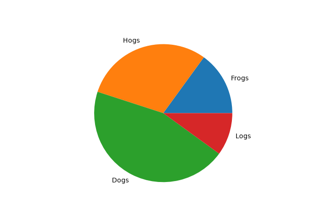Basic pie chart

Basic pie chart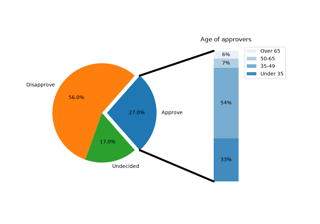Bar of pie

Bar of pie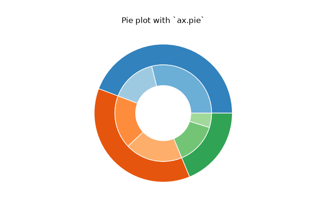Nested pie charts

Nested pie charts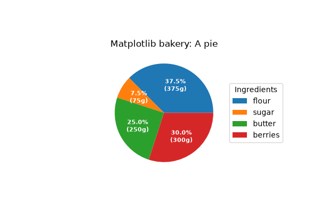Labeling a pie and a donut

Labeling a pie and a donut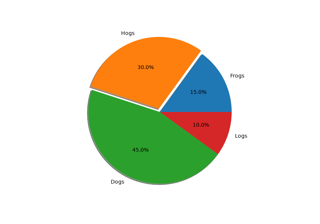SVG Filter Pie

SVG Filter Pie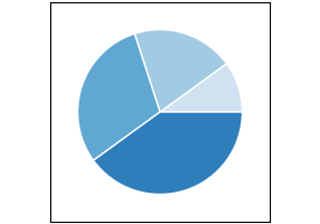pie(x)

pie(x)